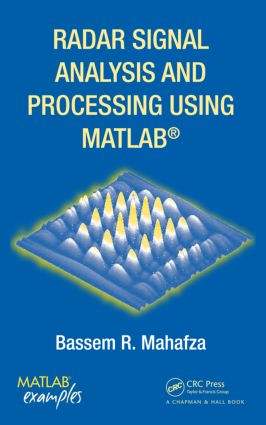Radar Signal Analysis and Processing Using MATLAB

1st Edition

Chapman and Hall/CRC

504 pages | 204 B/W Illus.

Hardback: 9781420066432
pub: 2008-06-19
\$145.00
x
eBook (VitalSource) : 9780429149337
pub: 2016-04-19
from \$28.98

FREE Standard Shipping!

Description

Offering radar-related software for the analysis and design of radar waveform and signal processing, Radar Signal Analysis and Processing Using MATLAB® provides a comprehensive source of theoretical and practical information on radar signals, signal analysis, and radar signal processing with companion MATLAB® code.

After an overview of radar systems operation and design, the book reviews elements of signal theory relevant to radar detection and radar signal processing, along with random variables and processes. The author then presents the unique characteristic of the matched filter and develops a general formula for the output of the matched filter that is valid for any waveform. He analyzes several analog waveforms, including the linear frequency modulation pulse and stepped frequency waveforms, as well as unmodulated pulse-train, binary, polyphase, and frequency codes. The book explores radar target detection and pulse integration, emphasizing the constant false alarm rate. It also covers the stretch processor, the moving target indicator, radar Doppler processing, beamforming, and adaptive array processing.

Using configurable MATLAB code, this book demonstrates how to apply signal processing to radar applications. It includes many examples and problems to illustrate the practical application of the theory.

Preface

Range Measurements

Range Resolution

Doppler Frequency

Coherence

Noise Figure

Effects of the Earth’s Surface on the Radar Equation

Atmospheric Attenuation

MATLAB Program Listings

Linear Systems and Complex Signal Representation

Signal and System Classifications

The Fourier Transform

Systems Classification

Signal Representation Using the Fourier Series

Convolution and Correlation Integrals

Bandpass Signals

Spectra of a Few Common Radar Signals

Signal Bandwidth and Duration

Discrete Time Systems and Signals

Random Variables and Processes

Random Variable

Multivariate Gaussian Random Vector

Rayleigh Random Variables

The Chi-Square Random Variables

Random Processes

Bandpass Gaussian Random Process

The Matched Filter

The Matched Filter SNR

Mean and Variance of the Matched Filter Output

General Formula for the Output of the Matched Filter

Waveform Resolution and Ambiguity

Range and Doppler Uncertainty

Target Parameter Estimation

The Ambiguity Function—Analog Waveforms

Introduction

Examples of the Ambiguity Function

Stepped Frequency Waveforms

Nonlinear FM

Ambiguity Diagram Contours

Interpretation of Range-Doppler Coupling in LFM Signals

MATLAB Programs and Function

The Ambiguity Function—Discrete Coded Waveforms

Discrete Code Signal Representation

Pulse-Train Codes

Phase Coding

Frequency Codes

Ambiguity Plots for Discrete Coded Waveforms

Target Detection and Pulse Integration

Target Detection in the Presence of Noise

Probability of False Alarm

Probability of Detection

Pulse Integration

Target Fluctuating

Probability of False Alarm Formulation for a Square Law Detector

Probability of Detection Calculation

Computation of the Fluctuation Loss

Cumulative Probability of Detection

Constant False Alarm Rate (CFAR)

MATLAB Program and Routines

Appendix: The Incomplete Gamma Function

Pulse Compression

Time-Bandwidth Product

Basic Principal of Pulse Compression

Correlation Processor

Stretch Processor

MATLAB Program Listings

Clutter Cross Section Density

Surface Clutter

Volume Clutter

Clutter RCS

Clutter Spectrum

Moving Target Indicator (MTI)

PRF Staggering

MTI Improvement Factor

Subclutter Visibility (SCV)

Delay Line Cancelers with Optimal Weights

MATLAB Program Listings

Doppler Processing

MATLAB Programs and Routines

Introduction

General Arrays

Linear Arrays

MATLAB Program Listings

Bibliography

Index

Problems appear at the end of each chapter.